Mobile QR Code1. (Dept. of Electronic and Electrical Eng., Ewha Womans University, Seoul, Korea)
2. (Graduate Program in Smart Factory, Ewha Womans University, Seoul, Korea)
3. (Post-Silicon Semiconductor Institute, Korea Institute of Science and Technology, Seoul, Korea)

CMOS, cross-coupled, feedforward, optoelectronic, TIA

## I. INTRODUCTION

For the past decade, LiDAR sensors have received a great deal of attention because they can be applied to various industries including unmanned vehicles, mobile robots, mobile phones, home-monitoring systems, etc. Particularly, short-range LiDAR sensors can be exploited to provide an efficient solution for real-time detection of falling accidents in long-term care facilities (LCTF), where senile dementia patients frequently suffer from falls without recognition of nurses and sometimes face death in consequence. Therefore, it becomes more crucial to detect the sudden falls and convey the information to either nurses or families to avoid more perilous situations .

Most time-of-flight (ToF) LiDAR sensors are categorized to either Geiger mode or linear mode. The former utilizes single-photon avalanche diodes (SPAD) whereas the latter uses APDs . In conventional linear-mode ToF LiDAR sensors, a transmitter (Tx) emits light pulses whereas off-chip APDs in a receiver (Rx) detect the reflected light pulses and generate photocurrents. However, off-chip APDs are integrated on PC-boards by using bond-wires, which may however deteriorate the Rx bandwidth and noise performance considerably, not to mention the increase of packaging cost. Hence, in this work, on-chip APDs are realized in a 180-nm CMOS process to avoid the aforementioned issues in the design of a linear-mode LiDAR sensor.

Fig. 1 shows a simplified block diagram of a linear-mode LiDAR sensor, in which the Tx consists of a laser diode and driver and the Rx comprises an optoelectronic transimpedance amplifier (OTIA) and a time-to-digital converter (TDC) that initializes the range detection mechanism and thus determines the distance to targets. The proposed OTIA includes an on-chip APD, a voltage-mode feedforward input circuit (VFIC), a single-to-differential (S2D) converter, a cross-coupled inverter-based post-amplifier (CI-PA), and an output buffer (OB).

##### Fig. 1. Block diagram of a conventional LiDAR system.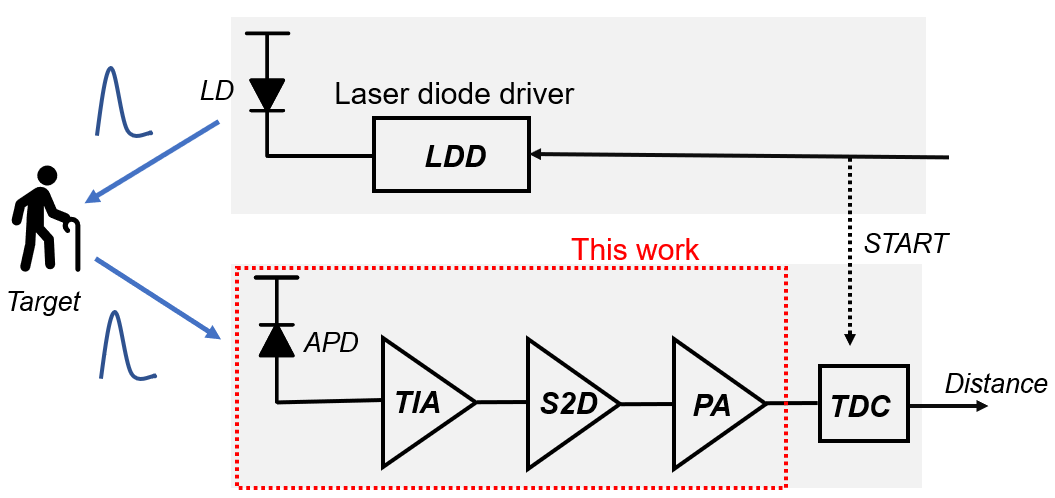This paper is organized as follows. Section II describes the realization of a P$^{+}$/N-well APD and the circuit design of OTIA. Section III demonstrates the measured results of the OTIA chip. Then, a conclusion is followed in Section IV.

## II. CIRCUIT DESCRIPTION

Fig. 2 depicts the block diagram of the proposed OTIA, consisting of an on-chip APD to generate photocurrents, a VFIC to change the incoming photocurrent pulses to voltage outputs, a S2D converter to yield differential signals for improved common-mode rejection ratio (CMRR), a 2$^{\mathrm{nd}}$ stage differential amplifier for boosting gain further, a CI-PA to reduce mismatches between differential pulses, and an OB for 50-${\Omega}$ impedance matching.

##### Fig. 2. Schematic diagram of the proposed optoelectronic transimpedance amplifier (OTIA).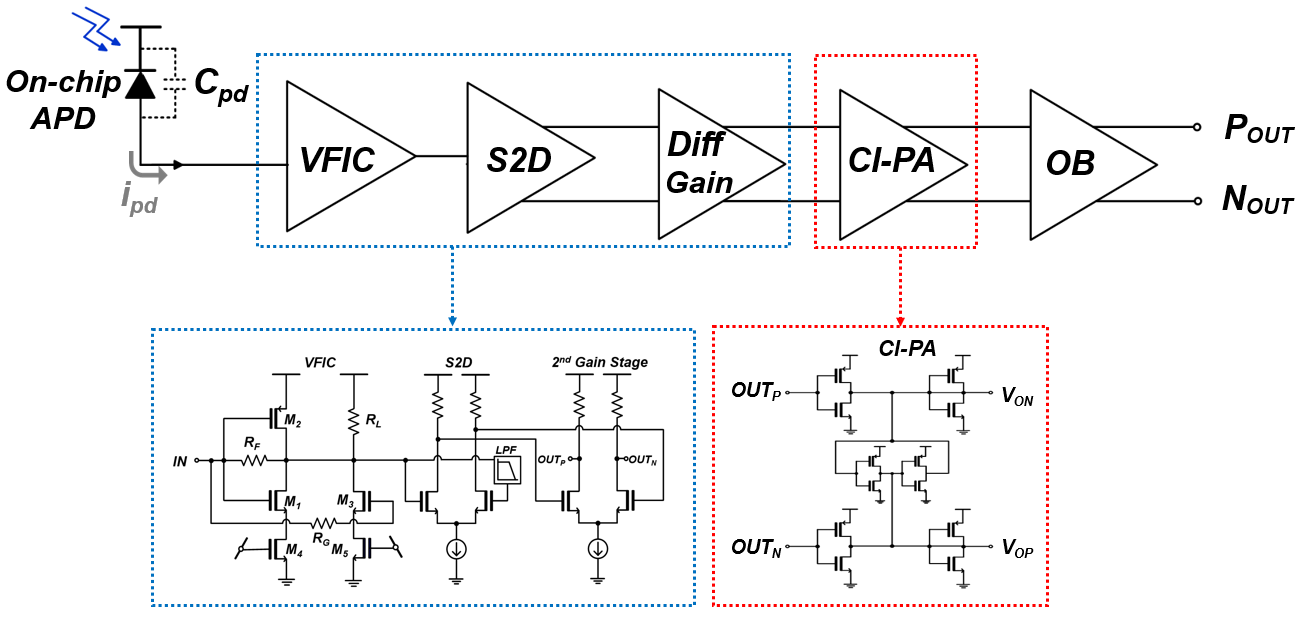### 1. P$^{+}$/N-well APD

Fig. 3 illustrates the cross-sectional view of the P$^{+}$/N-well APD realized in a 180-nm CMOS process, where charge neutron region is small due to the narrow N-well region. Therefore, holes can participate in avalanche process after short diffusion time, hence leading to the extended bandwidth. The measured results of the on-chip P+/N-well are the same as those demonstrated in .

##### Fig. 3. Cross-sectional view of the on-chip P$^{+}$/N-well APD.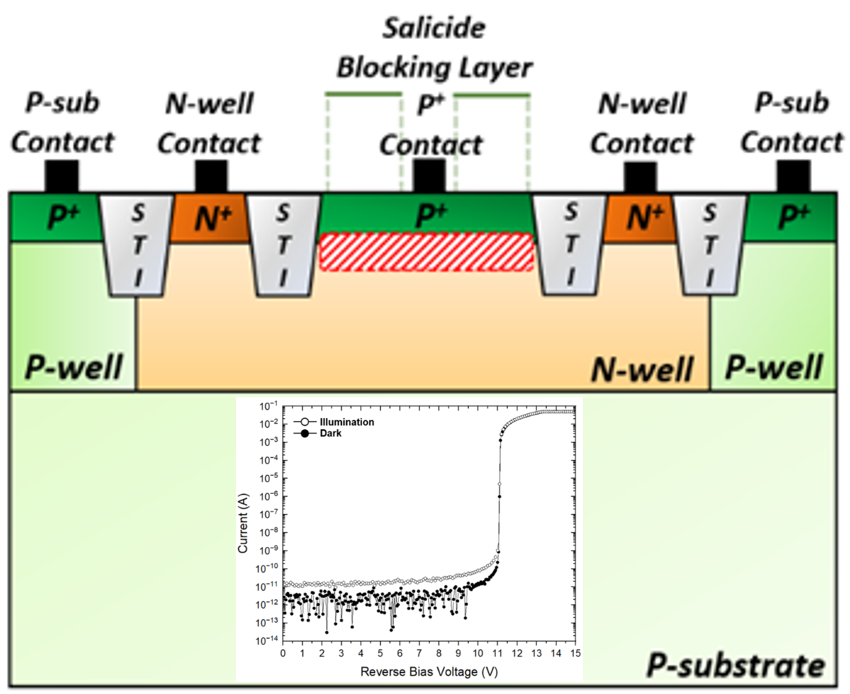### 2. Voltage-mode Feedforward Input Circuit

Fig. 4 shows the schematic diagram of the VFIC, in which a voltage-mode inverter (INV) input stage with a feedback resistor (R$_{\mathrm{F}}$) is merged with a feedforward common-source amplifier of which gate node is connected to the gates of the INV input stage .

##### Fig. 4. Schematic diagram of the VFIC block.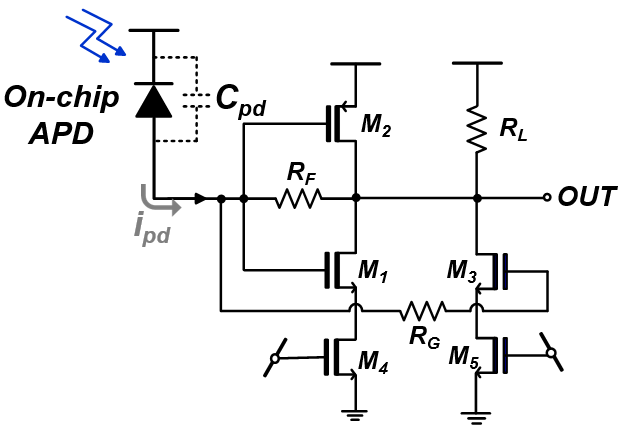Small signal analysis shows that the input resistance of the VFIC is given by,

##### (1)
$R_{in}\cong \frac{1+\left(3R_{F}/ro\right)}{g_{m1}+g_{m2}+g_{m3}}\cong \frac{1}{g_{m1}+g_{m2}+g_{m3}}$

, where g$_{\mathrm{m(i=1\sim 3)}}$ and r$_{\mathrm{o(i=1\sim 3)}}$ represent the transconductance and the output resistance of transistors (M$_{1}$\textasciitilde{}M$_{3}$), respectively. It is noted that the drain nodes of M$_{4}$ and M$_{5}$ are assumed to be AC-grounded for simplicity.

The mid-band transimpedance gain is given by,

##### (2)
\begin{aligned} Z_T(0) &=-\frac{\left(g_{m 1}+g_{m 2}+g_{m 3}\right) R_F-1}{g_{m 1}+g_{m 2}+g_{m 3}+\frac{1}{r_{o 1}\left\|r_{o 2}\right\| r_{o 3} \| R_L}} \\ & \cong-R_F \end{aligned}

The input referred noise current spectral density of the VFIC is given by,

##### (3)
\begin{aligned} &{\overline{I^2}}_{n, T I A}(f) \cong \frac{4 k T}{R_F}+4 k T \Gamma\left(\frac{1}{g_{m 1}}+\frac{1}{g_{m 2}}\right) \\ &\quad \times\left[\left(2 \pi C_T\right)^2 f^2+\frac{1}{R_F^2}\right] \\ &\quad+4 k T\left(\Gamma \frac{1}{g_{m 3}}+R_G\right) \times\left[\left(2 \pi C_T\right)^2 f^2+\frac{1}{R_F^2}\right] \\ &\cong \frac{4 k T}{R_F}+4 k T \Gamma\left(\frac{1}{g_{m 1}}+\frac{1}{g_{m 2}}+\frac{1}{g_{m 3}}+R_G\right) \times\left(2 \pi C_T\right)^2 f^2 \end{aligned}

, where k is the Boltzmann’s constant, T is the absolute temperature, and $\Gamma \left(\approx 2\right)$ is the Ogawa’s noise factor of a MOSFET. Also, C$_{\mathrm{T}}$(= C$_{\mathrm{D}}$ + C$_{\mathrm{in,M1}}$+ C$_{\mathrm{in,M2}}$) represents the total capacitance at the input node of the VFIC, which includes the photodiode capacitance (C$_{\mathrm{D}}$) and the input capacitance of the INV input stage, i.e., C$_{\mathrm{in,M1}}$+ C$_{\mathrm{in,M2}}$ = C$_{\mathrm{gs1}}$+ C$_{\mathrm{gs2}}$+ (1+A$_{\mathrm{v}}$)·(C$_{\mathrm{gd1}}$+ C$_{\mathrm{gd2}}$).

Under the assumption that the VFIC has the maximally flat response (i.e., Q = 1/$\sqrt{2}$), the noise bandwidth (f$_{\mathrm{n1}}$) for white noise is 1.11 times the signal bandwidth (f$_{\mathrm{-3dB}}$), whereas the noise bandwidth (f$_{\mathrm{n2}}$) for f$^{2}$-noise is 1.49 times f$_{\mathrm{-3dB}}$ .

Then, the input-referred noise current of the VFIC is given by,

##### (4)
$\overline{i^{2}}_{n,\,TIA}\cong \frac{4kT}{R_{F}}f_{n1}+\frac{4kT\Gamma }{3}C_{T}^{2}f_{n2}^{2}\left(\frac{1}{g_{m1}}+\frac{1}{g_{m2}}+\frac{1}{g_{m3}}\right)$

Post-layout simulations were conducted by using the model parameters of the 180-nm CMOS RF G process. Fig. 5 shows the simulation results of the OTIA, where the transimpedance gain of 87.4 dB${\Omega}$, the bandwidth of 634 MHz, and the noise current spectral density of 5.69 pA/${\sqrt{}}$Hz are obtained.

##### Fig. 5. Simulated frequency response of the OTIA.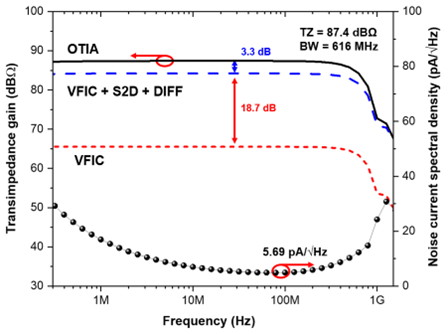### 3. Cross-coupled Inverter-based Post-amplifier

Fig. 6 depicts the schematic diagram of the CI-PA that consists of four inverters and two diode-connected output buffers. Thereby, the output voltages (V$_{ON}$ & V$_{OP}$) can be enhanced by merging the input signals (OUT$_{N}$ & OUT$_{P}$) with other small portions of another path (g$_{\mathrm{mb}}$). Provided that the value of g$_{\mathrm{ma}}$ is 4 times larger than that of g$_{\mathrm{mb}}$, the amplitude and phase mismatches can be reduced considerably .

##### Fig. 6. Schematic diagram of the CI-PA.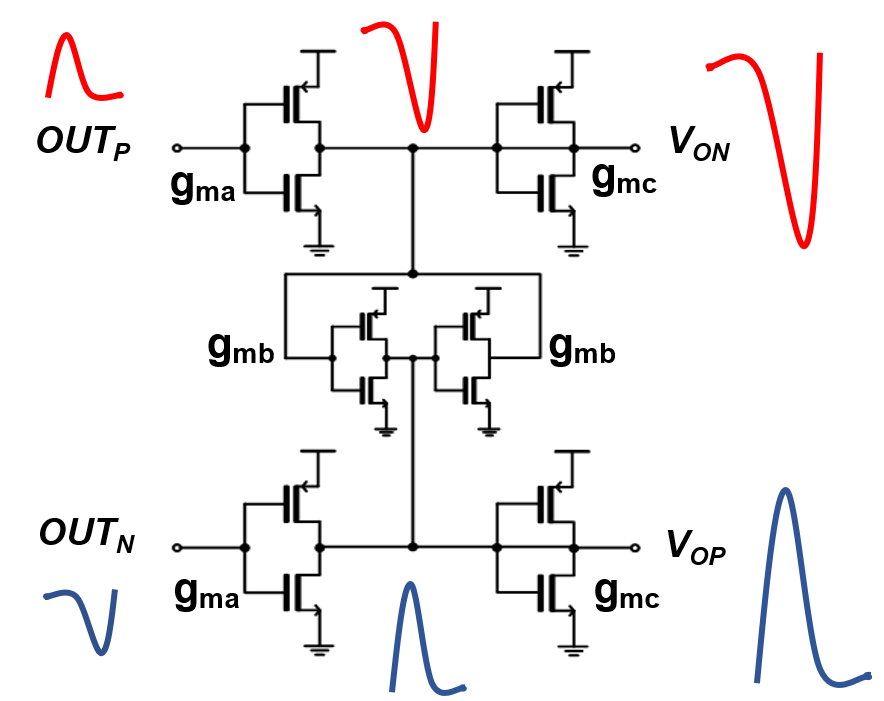It should be noted that this CI-PA still introduces amplitude mismatches between two outputs in the cases of short-distance detection because g$_{\mathrm{ma}}$ may vary severely with respect to the variation of v$_{\mathrm{gs}}$. Therefore, circuit design should be carefully conducted to match $\partial g_{man}/\partial v_{gs}$ with $\partial g_{map}/\partial v_{gs}$, where g$_{\mathrm{man}}$ and g$_{\mathrm{map}}$ represent the transconductance of NMOS and PMOS at the input inverter stage, respectively.

Post-layout simulations show that the CI-PA achieves the voltage gain of 3.3 dB and the bandwidth of 1.67 GHz. Fig. 7 compares the simulated pulse responses with and without the CI-PA for varying input current pulses up to 50 ${\mu}$A$_{\mathrm{pp}}$, where each pulse has 5-ns pulse width and 1-ns rise or fall duration. It is clearly observed that the CI-PA helps to improve the symmetry of output voltage pulses and to reduce the mismatch characteristics.

##### Fig. 7. Simulated pulse response with different input currents: (a) with CI-PA; (b) without CI-PA.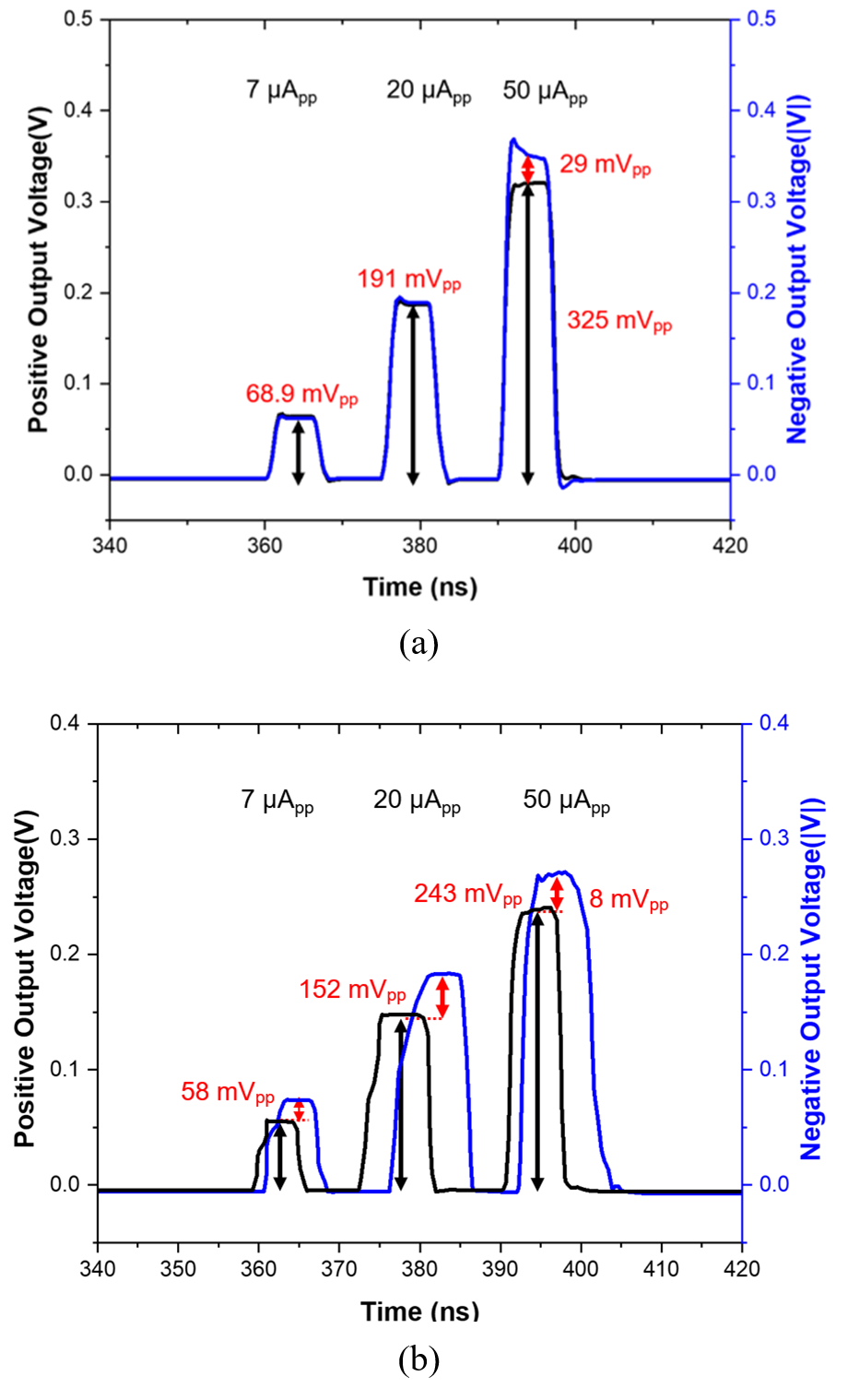## III. MEASURED RESULTS

Fig. 8 shows the chip micro-photograph of the proposed OTIA, in which the chip core occupies the area of 0.068 mm$^{2}$, and the whole chip occupies the area of 1.1${\times}$2.0 mm$^{2}$, including I/O pads. DC measurements reveal that the chip dissipates 39.3 mW.

##### Fig. 8. Chip micro-photograph of the OTIA.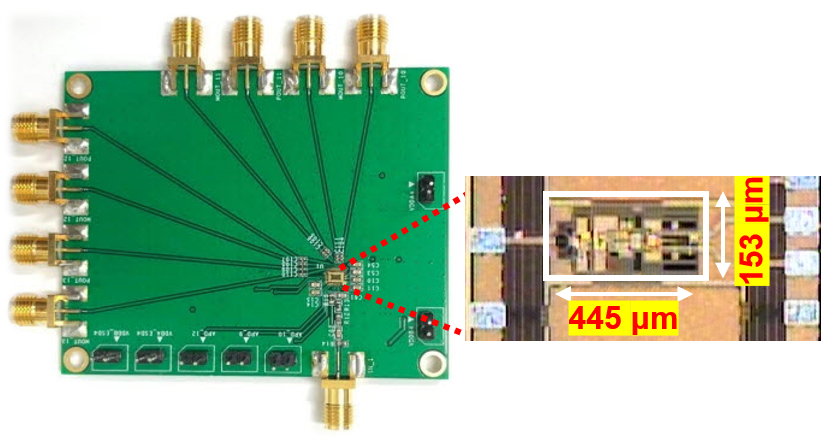For electrical measurements, the photodiode was emulated by its electrical lumped-model with a 25 ${\Omega}$ series resistor and a 500-fF parasitic capacitance. Fig. 9 demonstrates the measured S-parameters and the frequency response of the OTIA, respectively. Fig. 9(a) depicts the S$_{21}$ of 27 dB, the S$_{11}$ and S$_{22}$ of less than -10 dB within the bandwidth, and the S$_{12}$ of less than -30 dB. These S-parameters are transformed to Z-parameters by using the equation below .

##### (5)
$Z_{21}=\frac{2S_{21}}{\left(1-S_{11}\right)\left(1-S_{22}\right)-S_{21}S_{12}}$

Fig. 9(b) reveals the transimpedance gain of 95.1 dB${\Omega}$, and the bandwidth of 608 MHz with the equivalent input resistance 63.3 ${\Omega}$.

##### Fig. 9. Measured: (a) S-parameters; (b) frequency response of the OTIA.Fig. 10 shows the measured output noise voltage of the OTIA, where the background noise of the utilized oscilloscope (Agilent DCA 86100D) was considered. Then, the input referred average noise current spectral density is given by,

##### Fig. 10. Measured noise of the OTIA.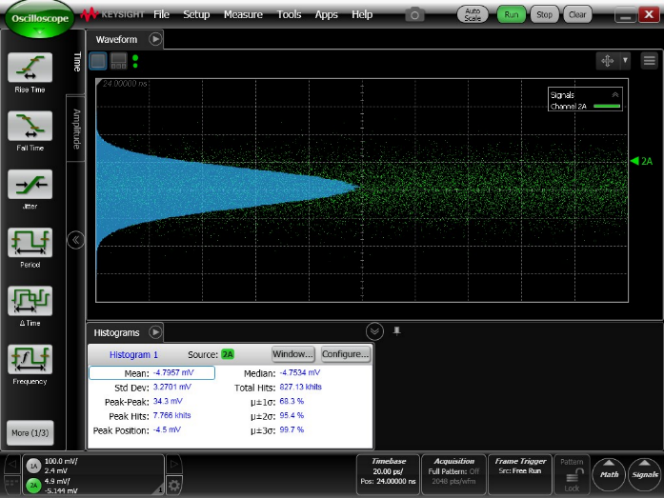##### (6)
$$I_{n, i n} \equiv \frac{2 \sqrt{(3.27 m V)^2-(0.75 \mathrm{mV})^2}}{95.1 \mathrm{dB \Omega}}=112 n A_{r m}$$
##### (7)
$$I_{n, i n, a r g} \equiv \frac{I_{n, \text { in }}}{\sqrt{608 M H_z}}=4.54 p A / \sqrt{H z}$$

The discrepancy from the simulation results may be attributed to the increased transimpedance gain.

This input-referred RMS noise current of 0.12 ${\mu}$A$_{\mathrm{RMS}}$ leads to the optical sensitivity of -29.5 dBm for bit-error-rate (BER) of 10$^{-12}$ which can satisfy the SNR of 14 in maximum. Thereby, it is translated to the minimum detectable signal (MDS) of 2.38 ${\mu}$A$_{\mathrm{pp}}$ since the signal-to-noise ratio (SNR) of 10 is usually required for successful detection in LiDAR systems .

Fig. 11 demonstrates the measured eye-diagrams of the OTIA at 500 Mb/s with different input currents of 20~${\mu}$A$_{\mathrm{pp}}$ and 50 ${\mu}$A$_{\mathrm{pp}}$, respectively, in which a singled-ended output was measured with a 50-${\Omega}$ termination. Hence, each output amplitude corresponds to 91.6 dB${\Omega}$ and 85.2 dB${\Omega}$ transimpedance gain, respectively.

##### Fig. 11. Measured eye-diagrams of the OTIA for 2$^{31}$-1 PRBS input currents at 500-Mb/s data rates: (a) 20 ${\mu}$A$_{\mathrm{pp}}$; (b) 50 ${\mu}$A$_{\mathrm{pp}}$, respectively.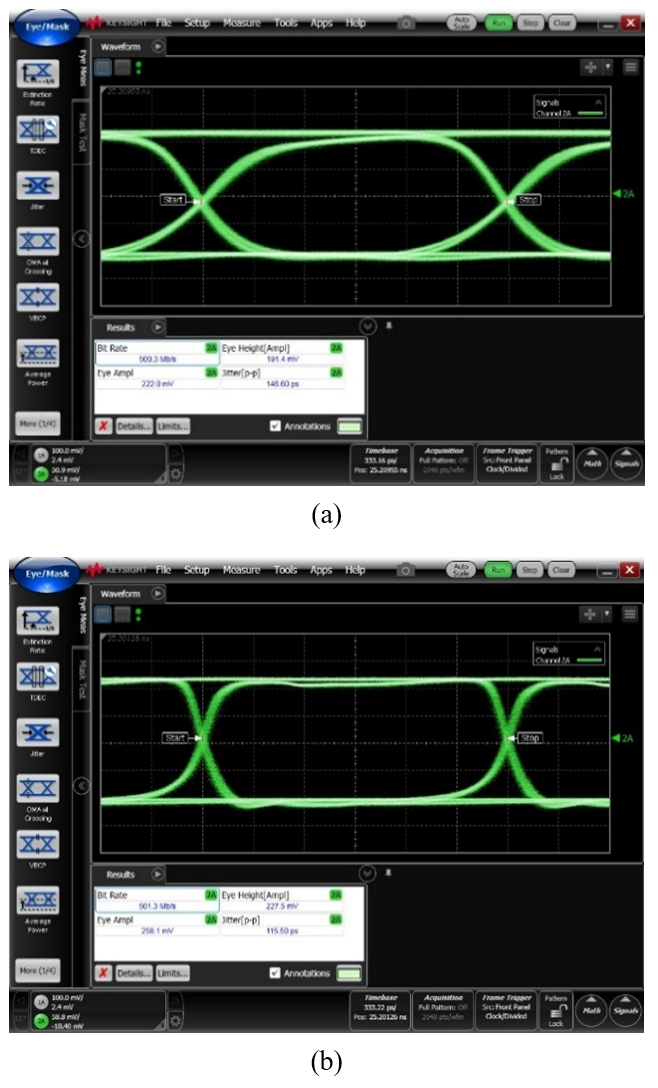Table 1 summarizes the performance of the proposed OTIA with the previously reported CMOS TIAs. Ref.  suggested a CMOS optoelectronic receiver IC with an on-chip APD for home-monitoring LiDAR sensors. Nonetheless, it revealed poorly recovered optical pulses with 8-mV$_{\mathrm{pp}}$ amplitude and 25-ms pulse width. Ref.  demonstrated a voltage-mode TIA, achieving a very high transimpedance gain. Yet, it required AC coupling capacitors and bias resistors for the interconnection between the off-chip APD and the receiver IC. Ref.  introduced a frequency compensation method onto a shunt feedback TIA, thereby improving the phase margin. However, the additional frequency compensation was required because of the exploited off-chip photodiode that mandates a bond wire between photodiode and the receiver chip, i.e., a critical shortcoming. Ref.  presented a linear receiver array consisting of 16 TIAs with low power, low noise, high transimpedance gain and wide bandwidth characteristics. Yet, it revealed poor dynamic range owing to its high transimpedance gain.

##### Table 1. Performance Comparison with Previously Reported CMOS TIAs for LiDAR Applications
 Parameters     This work CMOS technology (nm) 180 350 180 180 180 Supply (V) 1.8 3.3 3.3 1.8/3.3 1.8 APD On-chip Off-chip Off-chip Off-chip On-chip Input configuration VCF SF SF with FC SF VCF Bandwidth (MHz) 790 230 281 450 608 TZ gain (dB${\Omega}$) 93.4 100 86 100 95.1 Noise current spectral density (pA/sqrt(Hz)) 12 6.32 4.68 2.59 4.54 Min. detectable current (uA$_{\mathrm{pp}}$) 6.74 (SNR = 10) 1.0 (SNR = 5) 2.0 (SNR = 25) 2.5 (SNR = 5) 2.38 (SNR = 10) Power dissipation (mW) 56.5 180 200 6.6 39.3 Core area (㎟) 0.09 14 2.20 4.08 0.068
VCF: voltage-mode CMOS feedforward, SF: shunt feedback, FC: frequency compensation, SNR: signal-to-noise ratio

In this work, we have optimized the input VFIC integrated with on-chip APD and exploited a CI-PA to boost the output voltages further. Also, the circuit simplicity of the CI-PA helps to reduce the noise current spectral density for a comparable power dissipation characteristic Hence, this work shows better noise current spectral density and the corresponding minimum detectable current characteristics than the results demonstrated especially in Ref. .

## IV. CONCLUSIONS

We have realized an optoelectronic voltage-mode TIA consisting of an on-chip APD, the VFIC input stage, and the CI-PA. The design optimization renders the proposed OTIA to} provide a high-gain, low-noise, low-cost solution for short-range LiDAR sensors.

## ACKNOWLEDGMENTS

This work was supported by the National Research Foundation of Korea (NRF) grant funded by the Korea government (MSIT) (No. 2020R1A2C1008879). This research was supported by the MSIT (Ministry of Science, ICT), Korea, under the High-Potential Individuals Global Training Program) (2021-0-02133) supervised by the IITP (Institute for Information & Communications Technology Planning & Evaluation). This work was supported by the National Research Foundation (NRF), Korea, under project BK21 FOUR. The EDA tool and chip fabrication were supported by the IC Design Education Center.

## References

1
Yoon D., et al. , 2021, Mirrored Current-Conveyor Transimpedance Amplifier for Home Monitoring LiDAR Sensors, IEEE Sensors J., Vol. 21, pp. 5589-55972
Hong C., et al. , Sep. 2018, A Linear-Mode LiDAR Sensor Using a Multi-Channel CMOS Transimpedance Amplifier Array, IEEE Sensors J., Vol. 18, No. 17, pp. 7032-70403
Joo J. -E., et al. , Jun. 2021., A CMOS optoelectronic receiver IC with an on-chip APD for home-monitoring LiDAR sensors, Sensors, Vol. 21, No. 13, pp. 43644
Säckinger Eduard , 2005, Broadband circuits for Optical Fiber Communication. Hoboken, N.J: Wiley5
Kurtti S., Kostamovaara J., May 2020, A CMOS Receiver-TDC Chip Set for Accurate Pulsed TOF Laser Ranging, IEEE Trans. Instrum. Meas., Vol. 69, No. 5, pp. 2208-22176
Voinigescu S., 2013, High-frequency integrated circuits., Cambridge: Cambridge University Press7
Baharmast A., et al. , Aug. 2020, A Wide Dynamic Range Laser Radar Receiver Based on Input Pulse-Shaping Techniques, in IEEE Transactions on Circuits and Systems I: Regular Papers, Vol. 67, No. 8, pp. 2566-25778
Wang X., et al , Dec. 2020, A Low Walk Error Analog Front-End Circuit With Intensity Compensation for Direct ToF LiDAR, IEEE Trans. on Circuits and Systems I: Regular Papers, Vol. 67, No. 12, pp. 4309-43219
Zheng H., Ma R., Liu M., Zhu Z., 1 Jul. 2019, A Linear-Array Receiver Analog Front-End Circuit for Rotating Scanner LiDAR Application, IEEE Sensors J., Vol. 19, No. 13, pp. 5053-5061Yu Hu received the B.S. degree in electrical engineering and auto-mation from Anqing Normal University, Anqing, China, in 2016. She is currently pursuing the M.S. degree with the Analog Circuits and Systems Laboratory, Elwha Womans University. Her current research interests include silicon photonics, and CMOS optoelectronic integrated circuits and architectures for short distance optical application systems and sensor interface IC designs.

Ji-Eun Joo received the B.S. degree in electronic and electrical engi-neering from Ewha Womans University, Korea, in 2020. She is currently working toward the MSc degree in the analog circuits and systems lab. at the same university. Her current research interests include silicon photonics, and CMOS optoelectronic integrated circuits and architectures for short distance optical application systems and sensor interface IC designs.

Myung-Jae Lee received the B.S., M.S., and Ph.D. degrees in electrical and electronic engineering from Yonsei University, Seoul, South Korea, in 2006, 2008, and 2013, respectively. From 2013 to 2017, he was a Postdoctoral Researcher with the faculty of electrical engineering, Delft University of Technology (TU Delft), Delft, The Netherlands, and in 2017, he joined the school of engineering, École Polytechnique Fédérale de Lausanne (EPFL), Neuchâtel, Switzerland, as a Scientist. Since 2019, he has been a Principal Research Scientist with the Post-Silicon Semiconductor Institute, Korea Institute of Science and Technology (KIST), Seoul, South Korea, where he has led the research and development of next-generation single-photon detectors and sensors for various applications. His research interests include CMOS-compatible avalanche photodetectors and single-photon avalanche diodes and applications thereof.

Sung Min Park received the B.S. degree in electrical and electronic engineering from KAIST, Korea, in 1993. He received the M.S. degree in electrical engineering from University College London, U.K., in 1994, and the Ph.D. degree in electrical and electronic engineering from Imperial College London, U.K., in May 2000. In 2004, he joined the faculty of the Department of Electronics Engineering at Ewha Womans University, Seoul, Korea, where he is currently a Professor. His research interests include high-speed analog/digital integrated circuit designs in CMOS technologies for the applications of optical interconnects, silicon photonics, and LiDAR sensors.